Chapter 21 Electric Charge and Electric Field Copyright

• Slides: 55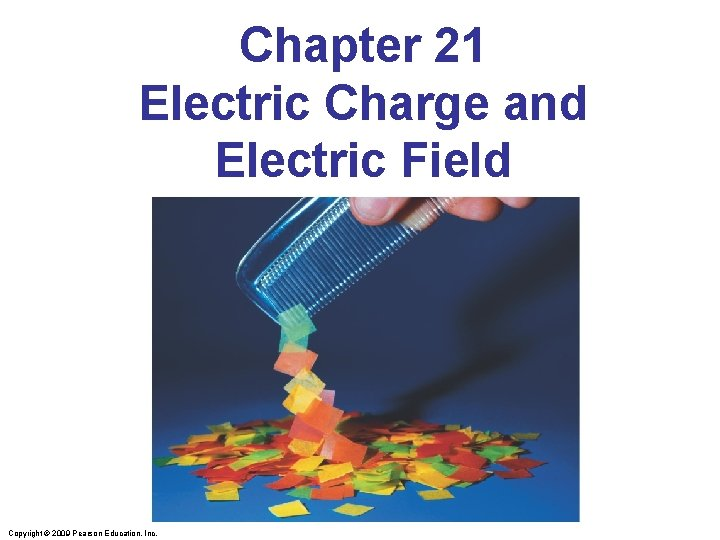Chapter 21 Electric Charge and Electric Field Copyright © 2009 Pearson Education, Inc.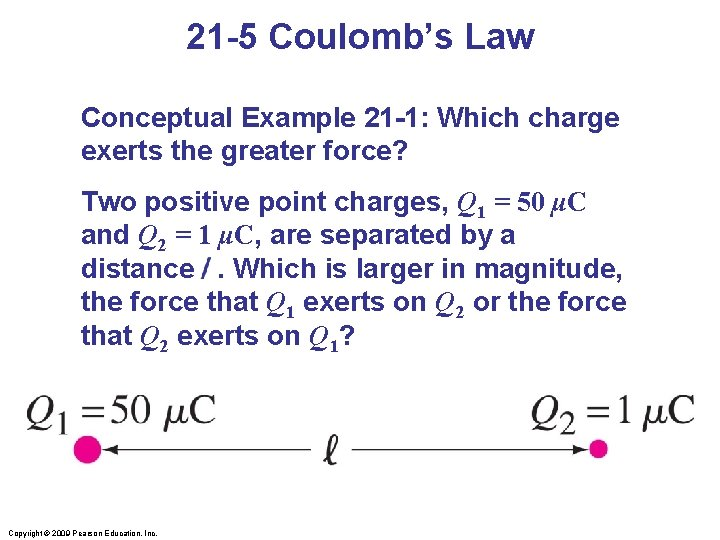21 -5 Coulomb’s Law Conceptual Example 21 -1: Which charge exerts the greater force? Two positive point charges, Q 1 = 50 μC and Q 2 = 1 μC, are separated by a distance. Which is larger in magnitude, the force that Q 1 exerts on Q 2 or the force that Q 2 exerts on Q 1? Copyright © 2009 Pearson Education, Inc.21 -5 Coulomb’s Law Example 21 -2: Three charges in a line. Three charged particles are arranged in a line, as shown. Calculate the net electrostatic force on particle 3 (the -4. 0 μC on the right) due to the other two charges. Copyright © 2009 Pearson Education, Inc.Concep. Test 21. 4 b Electric Force II Two balls with charges +Q and +4 Q are separated by 3 R. Where should you place another charged ball Q 0 on the line between the two charges such that the net force on Q 0 will be zero? +4 Q +Q 1 2 3 4 2 R R 3 R 5Concep. Test 21. 4 b Electric Force II Two balls with charges +Q and +4 Q are separated by 3 R. Where should you place another charged ball Q 0 on the line between the two charges such that the net force on Q 0 will be zero? +4 Q +Q 1 2 3 4 5 2 R R 3 R The force on Q 0 due to +Q is: F = k(Q 0)(Q)/R 2 The force on Q 0 due to +4 Q is: F = k(Q 0)(4 Q)/(2 R)2 Since +4 Q is 4 times bigger than +Q, Q 0 needs to be farther from +4 Q. In fact, Q 0 must be twice as far from +4 Q, since the distance is squared in Coulomb’s law.Concep. Test 21. 5 c Proton and Electron III A proton and an electron are held apart a distance of 1 m and then let go. Where would they meet? 1) in the middle 2) closer to the electron’s side 3) closer to the proton’s side p eConcep. Test 21. 5 c Proton and Electron III A proton and an electron are held apart a distance of 1 m and then let go. Where would they meet? 1) in the middle 2) closer to the electron’s side 3) closer to the proton’s side By Newton’s 3 rd law, the electron and proton feel the same force But, since F = ma, and since the proton’s mass is much greater, greater the proton’s acceleration will be much smaller! smaller p Thus, they will meet closer to the proton’s original position Follow-up: Which particle will be moving faster when they meet? e21 -5 Coulomb’s Law Example 21 -3: Electric force using vector components. Calculate the net electrostatic force on charge Q 3 shown in the figure due to the charges Q 1 and Q 2. Copyright © 2009 Pearson Education, Inc.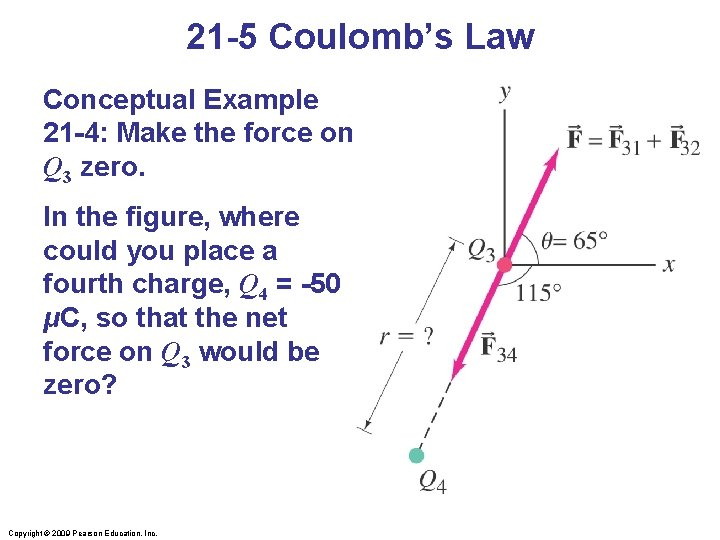21 -5 Coulomb’s Law Conceptual Example 21 -4: Make the force on Q 3 zero. In the figure, where could you place a fourth charge, Q 4 = -50 μC, so that the net force on Q 3 would be zero? Copyright © 2009 Pearson Education, Inc.21 -6 The Electric Field The electric field is defined as the force on a small charge, divided by the magnitude of the charge: Copyright © 2009 Pearson Education, Inc.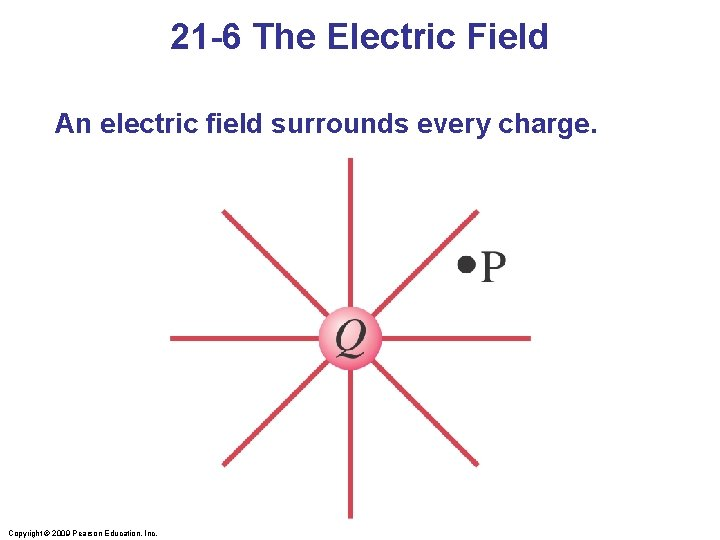21 -6 The Electric Field An electric field surrounds every charge. Copyright © 2009 Pearson Education, Inc.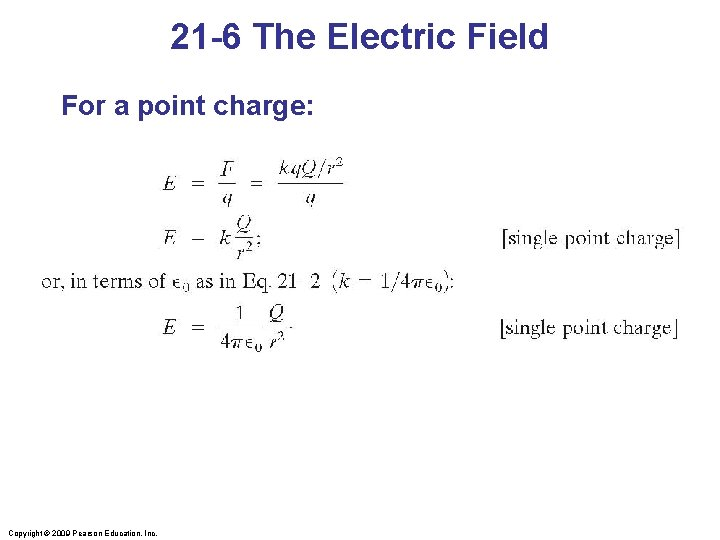21 -6 The Electric Field For a point charge: Copyright © 2009 Pearson Education, Inc.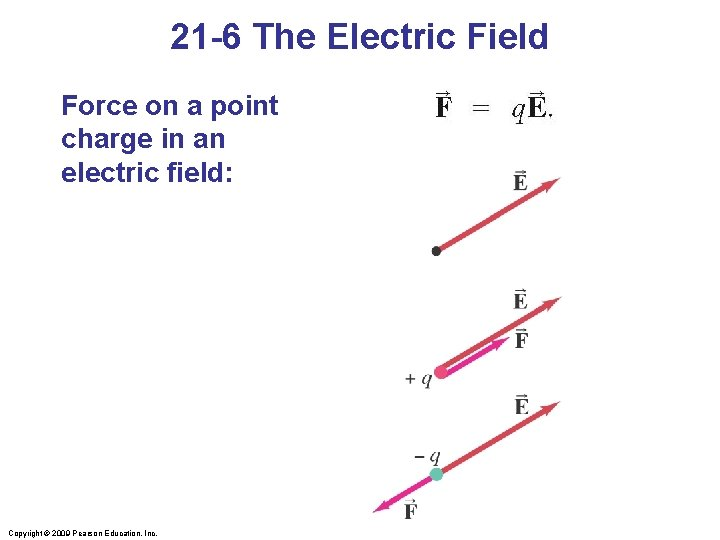21 -6 The Electric Field Force on a point charge in an electric field: Copyright © 2009 Pearson Education, Inc.21 -6 The Electric Field Example 21 -5: Photocopy machine. A photocopy machine works by arranging positive charges (in the pattern to be copied) on the surface of a drum, then gently sprinkling negatively charged dry toner (ink) particles onto the drum. The toner particles temporarily stick to the pattern on the drum and are later transferred to paper and “melted” to produce the copy. Suppose each toner particle has a mass of 9. 0 x 10 -16 kg and carries an average of 20 extra electrons to provide an electric charge. Assuming that the electric force on a toner particle must exceed twice its weight in order to ensure sufficient attraction, compute the required electric field strength near the surface of the drum. Copyright © 2009 Pearson Education, Inc.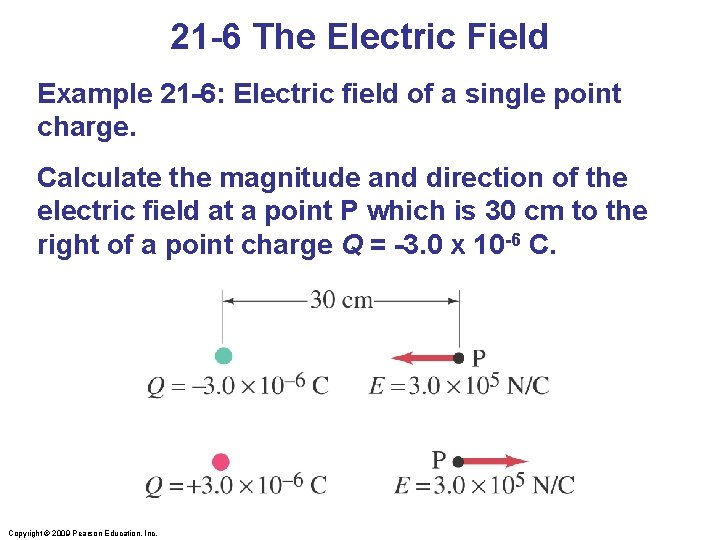21 -6 The Electric Field Example 21 -6: Electric field of a single point charge. Calculate the magnitude and direction of the electric field at a point P which is 30 cm to the right of a point charge Q = -3. 0 x 10 -6 C. Copyright © 2009 Pearson Education, Inc.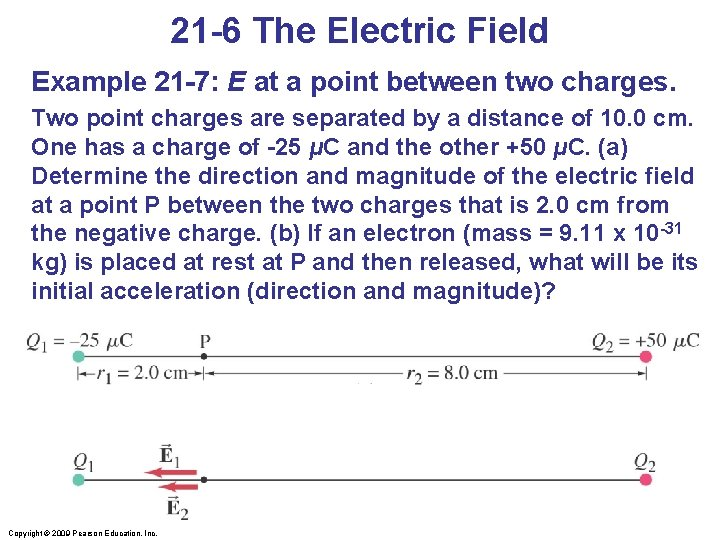21 -6 The Electric Field Example 21 -7: E at a point between two charges. Two point charges are separated by a distance of 10. 0 cm. One has a charge of -25 μC and the other +50 μC. (a) Determine the direction and magnitude of the electric field at a point P between the two charges that is 2. 0 cm from the negative charge. (b) If an electron (mass = 9. 11 x 10 -31 kg) is placed at rest at P and then released, what will be its initial acceleration (direction and magnitude)? Copyright © 2009 Pearson Education, Inc.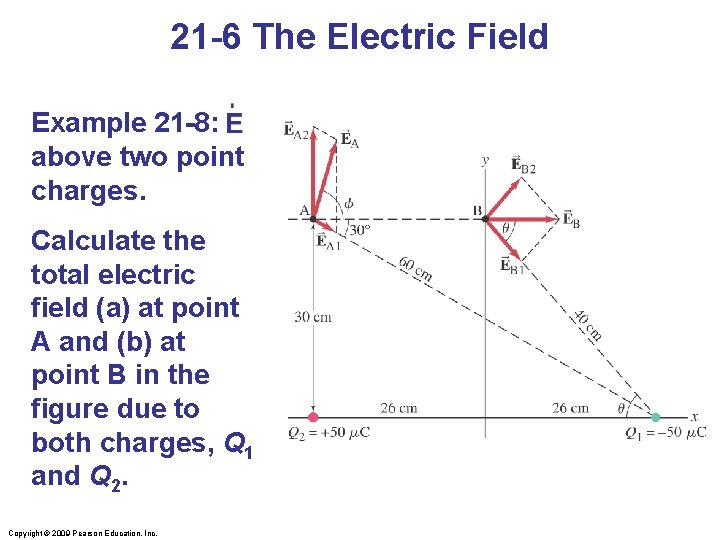21 -6 The Electric Field Example 21 -8: above two point charges. Calculate the total electric field (a) at point A and (b) at point B in the figure due to both charges, Q 1 and Q 2. Copyright © 2009 Pearson Education, Inc.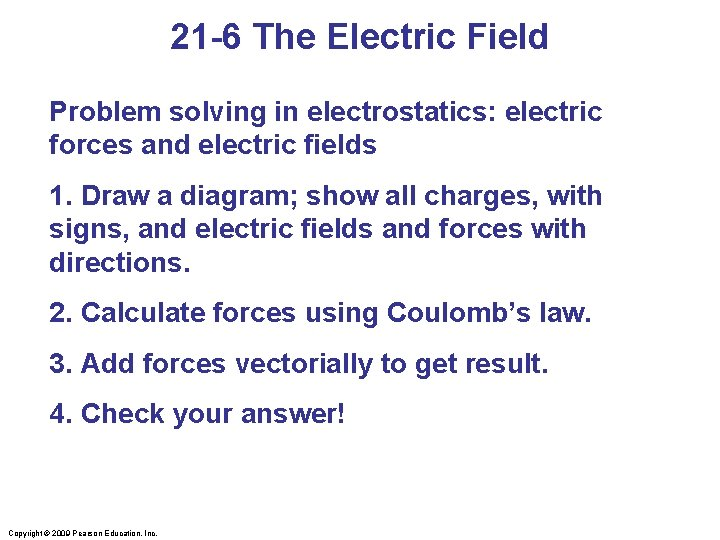21 -6 The Electric Field Problem solving in electrostatics: electric forces and electric fields 1. Draw a diagram; show all charges, with signs, and electric fields and forces with directions. 2. Calculate forces using Coulomb’s law. 3. Add forces vectorially to get result. 4. Check your answer! Copyright © 2009 Pearson Education, Inc.Concep. Test 21. 9 b Superposition II What is the electric field at -2 C 2 -2 C 1 the center of the square? 3 5) E = 0 -2 C 4 -2 C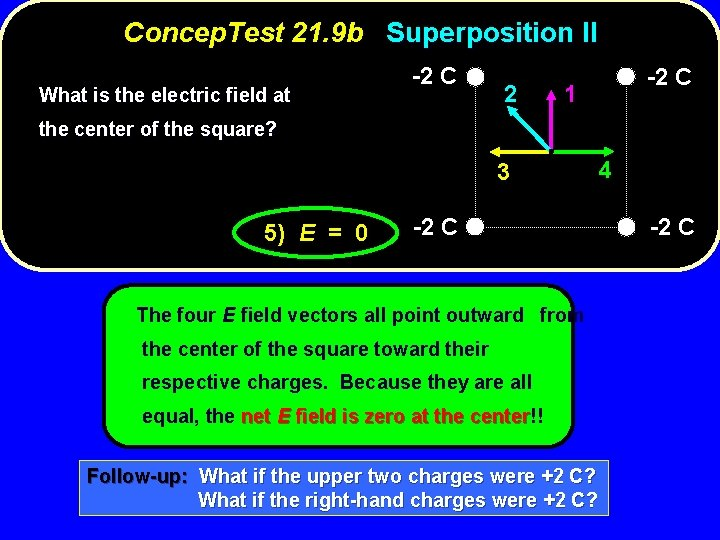Concep. Test 21. 9 b Superposition II What is the electric field at -2 C 2 -2 C 1 the center of the square? 3 5) E = 0 -2 C The four E field vectors all point outward from the center of the square toward their respective charges. Because they are all equal, the net E field is zero at the center!! center Follow-up: What if the upper two charges were +2 C? What if the right-hand charges were +2 C? 4 -2 C21 -7 Electric Field Calculations for Continuous Charge Distributions A continuous distribution of charge may be treated as a succession of infinitesimal (point) charges. The total field is then the integral of the infinitesimal fields due to each bit of charge: Remember that the electric field is a vector; you will need a separate integral for each component. Copyright © 2009 Pearson Education, Inc.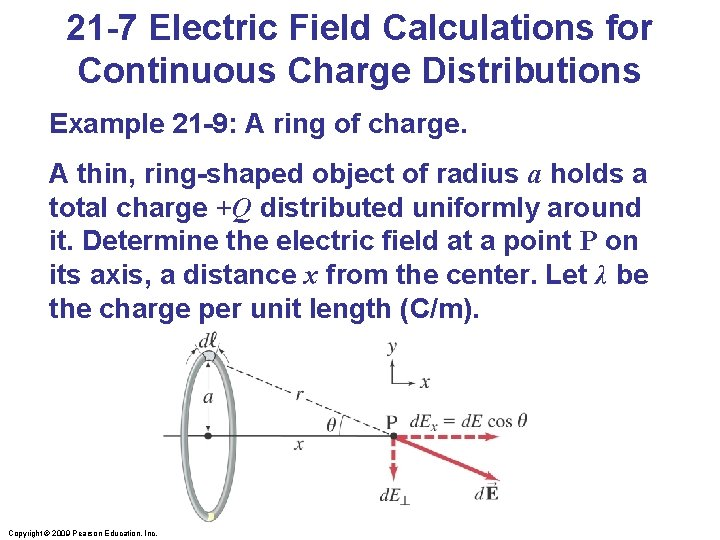21 -7 Electric Field Calculations for Continuous Charge Distributions Example 21 -9: A ring of charge. A thin, ring-shaped object of radius a holds a total charge +Q distributed uniformly around it. Determine the electric field at a point P on its axis, a distance x from the center. Let λ be the charge per unit length (C/m). Copyright © 2009 Pearson Education, Inc.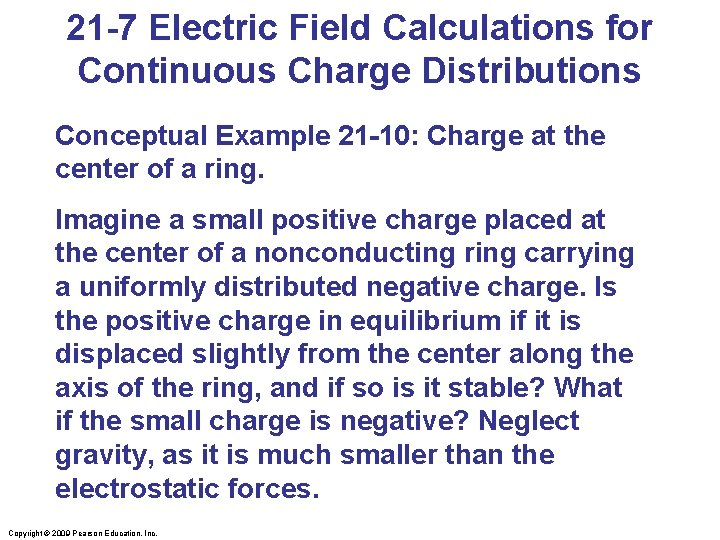21 -7 Electric Field Calculations for Continuous Charge Distributions Conceptual Example 21 -10: Charge at the center of a ring. Imagine a small positive charge placed at the center of a nonconducting ring carrying a uniformly distributed negative charge. Is the positive charge in equilibrium if it is displaced slightly from the center along the axis of the ring, and if so is it stable? What if the small charge is negative? Neglect gravity, as it is much smaller than the electrostatic forces. Copyright © 2009 Pearson Education, Inc.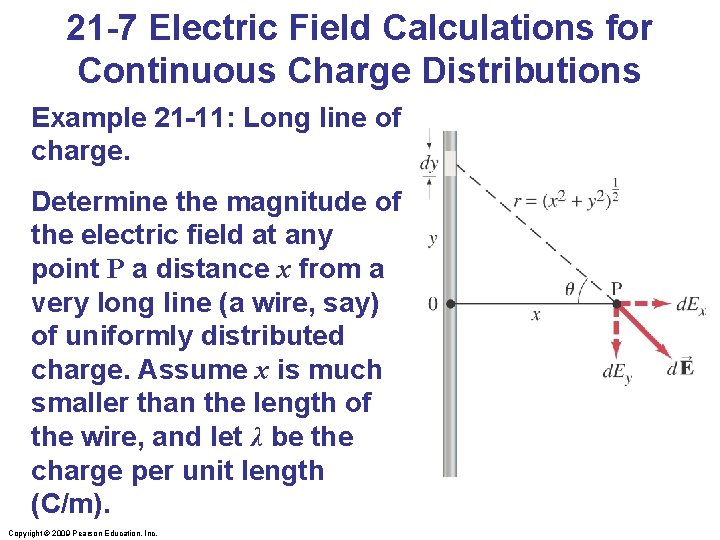21 -7 Electric Field Calculations for Continuous Charge Distributions Example 21 -11: Long line of charge. Determine the magnitude of the electric field at any point P a distance x from a very long line (a wire, say) of uniformly distributed charge. Assume x is much smaller than the length of the wire, and let λ be the charge per unit length (C/m). Copyright © 2009 Pearson Education, Inc.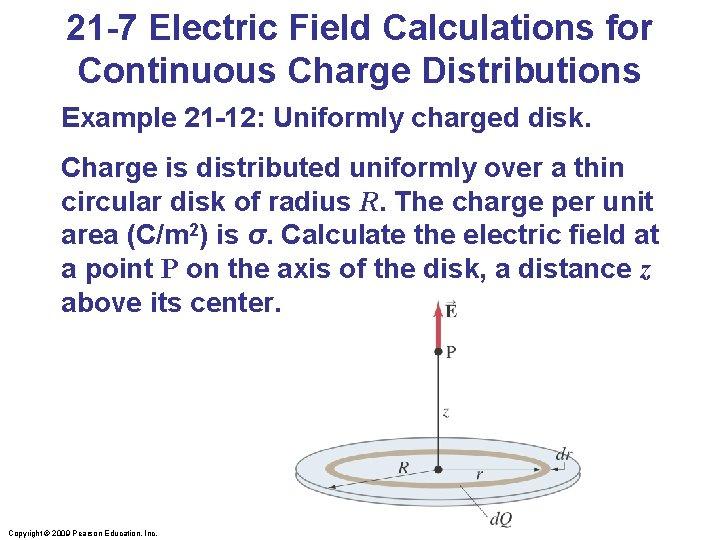21 -7 Electric Field Calculations for Continuous Charge Distributions Example 21 -12: Uniformly charged disk. Charge is distributed uniformly over a thin circular disk of radius R. The charge per unit area (C/m 2) is σ. Calculate the electric field at a point P on the axis of the disk, a distance z above its center. Copyright © 2009 Pearson Education, Inc.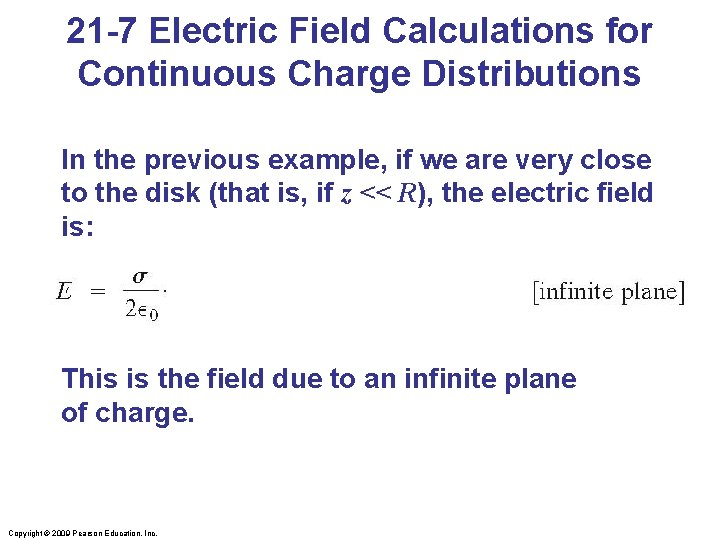21 -7 Electric Field Calculations for Continuous Charge Distributions In the previous example, if we are very close to the disk (that is, if z << R), the electric field is: This is the field due to an infinite plane of charge. Copyright © 2009 Pearson Education, Inc.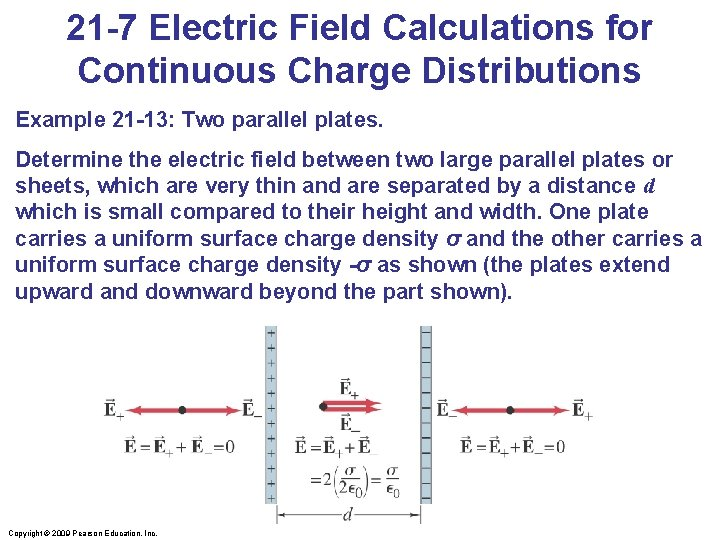21 -7 Electric Field Calculations for Continuous Charge Distributions Example 21 -13: Two parallel plates. Determine the electric field between two large parallel plates or sheets, which are very thin and are separated by a distance d which is small compared to their height and width. One plate carries a uniform surface charge density σ and the other carries a uniform surface charge density -σ as shown (the plates extend upward and downward beyond the part shown). Copyright © 2009 Pearson Education, Inc.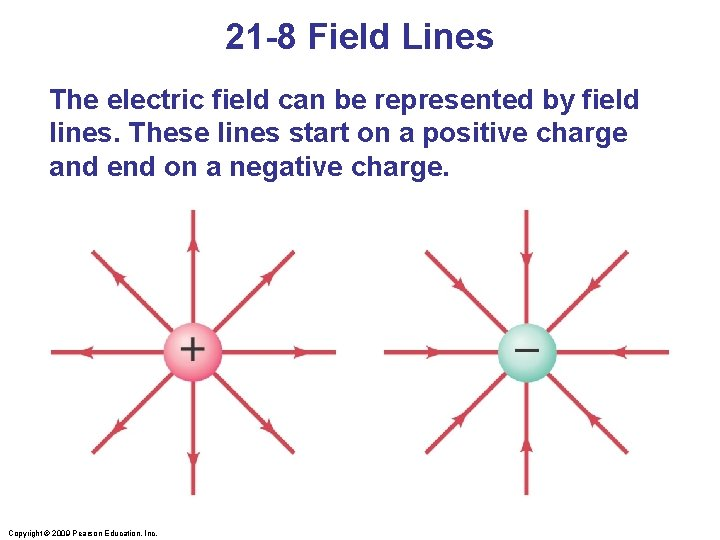21 -8 Field Lines The electric field can be represented by field lines. These lines start on a positive charge and end on a negative charge. Copyright © 2009 Pearson Education, Inc.21 -8 Field Lines The number of field lines starting (ending) on a positive (negative) charge is proportional to the magnitude of the charge. The electric field is stronger where the field lines are closer together. Copyright © 2009 Pearson Education, Inc.21 -8 Field Lines Electric dipole: two equal charges, opposite in sign: Copyright © 2009 Pearson Education, Inc.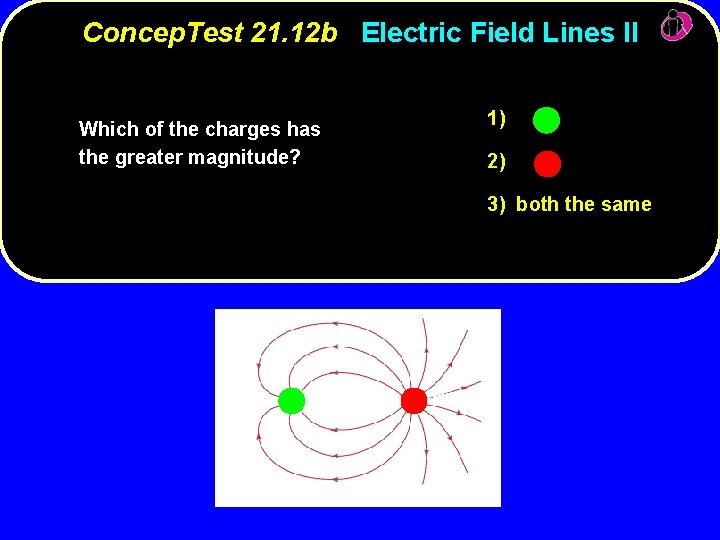Concep. Test 21. 12 b Electric Field Lines II Which of the charges has the greater magnitude? 1) 2) 3) both the same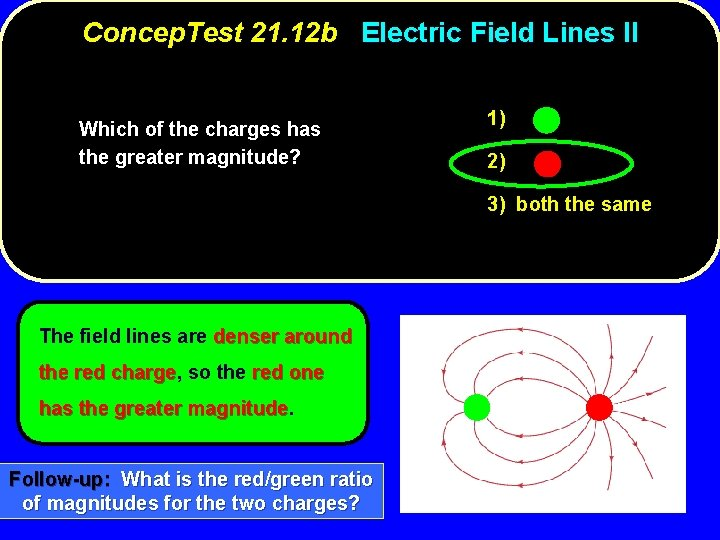Concep. Test 21. 12 b Electric Field Lines II Which of the charges has the greater magnitude? 1) 2) 3) both the same The field lines are denser around the red charge, charge so the red one has the greater magnitude Follow-up: What is the red/green ratio of magnitudes for the two charges?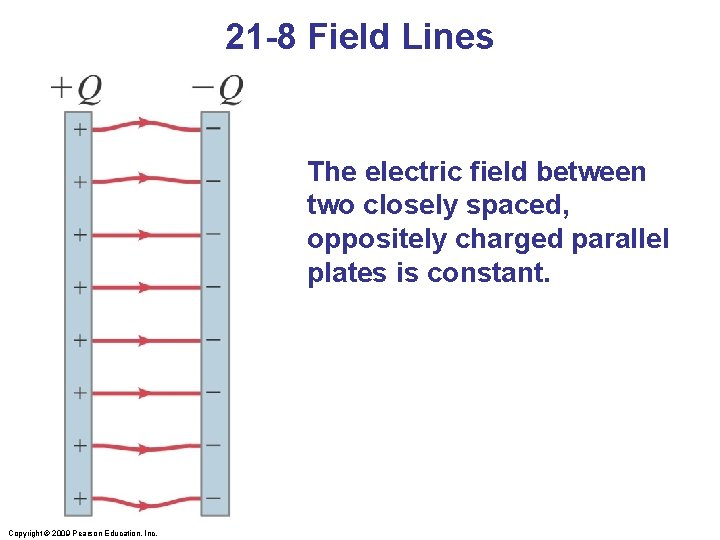21 -8 Field Lines The electric field between two closely spaced, oppositely charged parallel plates is constant. Copyright © 2009 Pearson Education, Inc.21 -8 Field Lines Summary of field lines: 1. Field lines indicate the direction of the field; the field is tangent to the line. 2. The magnitude of the field is proportional to the density of the lines. 3. Field lines start on positive charges and end on negative charges; the number is proportional to the magnitude of the charge. Copyright © 2009 Pearson Education, Inc.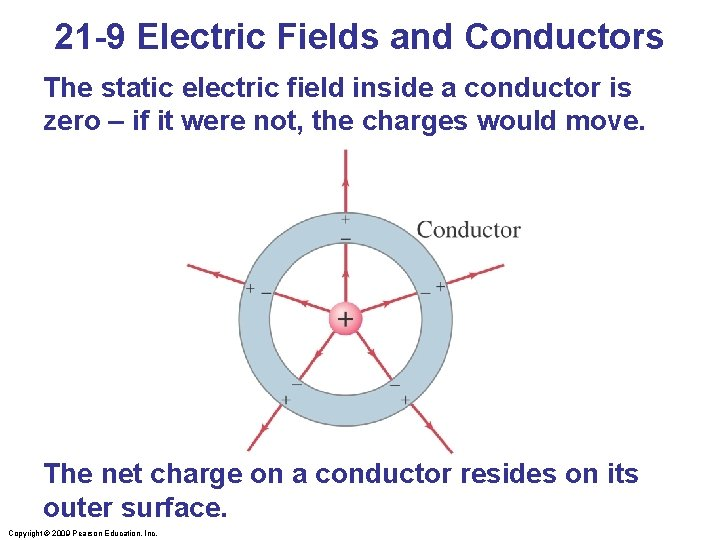21 -9 Electric Fields and Conductors The static electric field inside a conductor is zero – if it were not, the charges would move. The net charge on a conductor resides on its outer surface. Copyright © 2009 Pearson Education, Inc.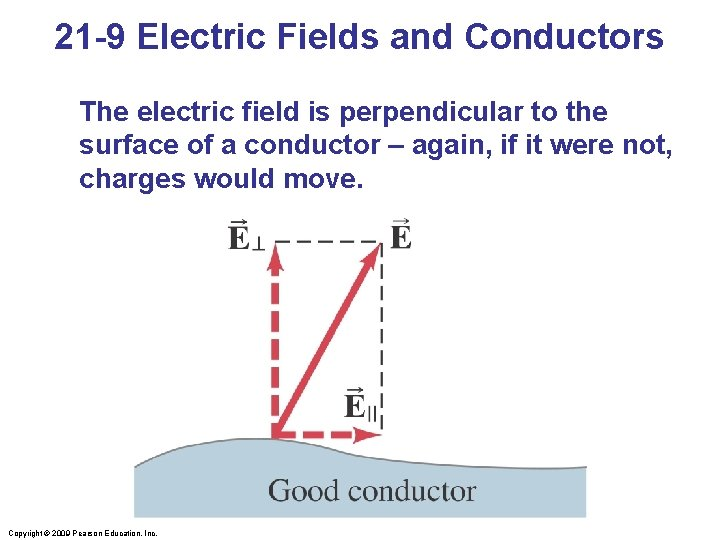21 -9 Electric Fields and Conductors The electric field is perpendicular to the surface of a conductor – again, if it were not, charges would move. Copyright © 2009 Pearson Education, Inc.21 -9 Electric Fields and Conductors Conceptual Example 21 -14: Shielding, and safety in a storm. A neutral hollow metal box is placed between two parallel charged plates as shown. What is the field like inside the box? Copyright © 2009 Pearson Education, Inc.21 -10 Motion of a Charged Particle in an Electric Field The force on an object of charge q in an electric field is given by: =q Therefore, if we know the mass and charge of a particle, we can describe its subsequent motion in an electric field. Copyright © 2009 Pearson Education, Inc.1 Concep. Test 21. 6 Forces in 2 D 2 3 Which of the arrows best 4 represents the direction of the net force on charge +2 Q d +Q +Q due to the other two charges? d +4 Q 5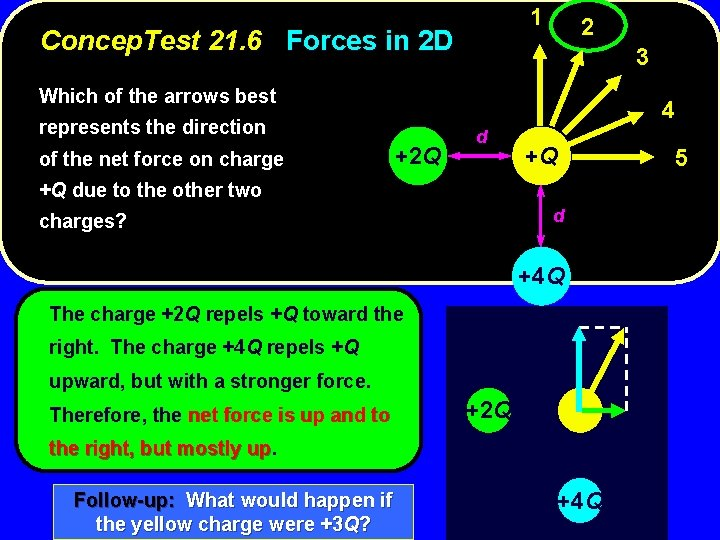1 Concep. Test 21. 6 Forces in 2 D 2 3 Which of the arrows best 4 represents the direction of the net force on charge +2 Q d +Q +Q due to the other two d charges? +4 Q The charge +2 Q repels +Q toward the right. The charge +4 Q repels +Q upward, but with a stronger force. Therefore, the net force is up and to +2 Q the right, but mostly up. up Follow-up: What would happen if the yellow charge were +3 Q? +4 Q 5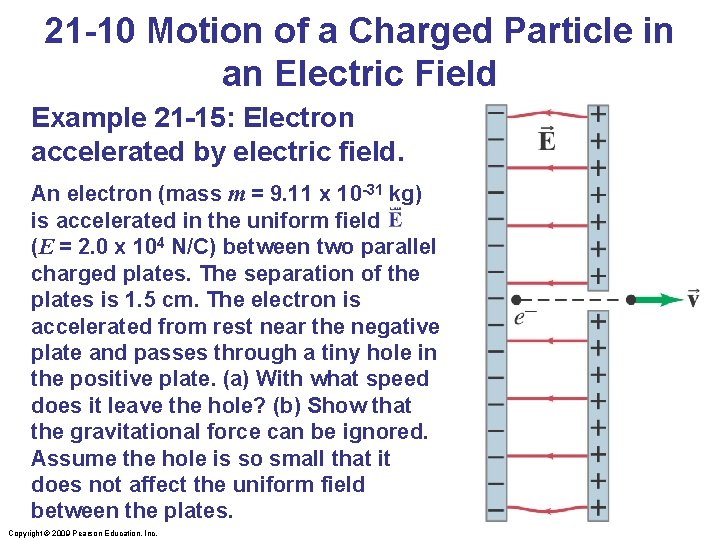21 -10 Motion of a Charged Particle in an Electric Field Example 21 -15: Electron accelerated by electric field. An electron (mass m = 9. 11 x 10 -31 kg) is accelerated in the uniform field (E = 2. 0 x 104 N/C) between two parallel charged plates. The separation of the plates is 1. 5 cm. The electron is accelerated from rest near the negative plate and passes through a tiny hole in the positive plate. (a) With what speed does it leave the hole? (b) Show that the gravitational force can be ignored. Assume the hole is so small that it does not affect the uniform field between the plates. Copyright © 2009 Pearson Education, Inc.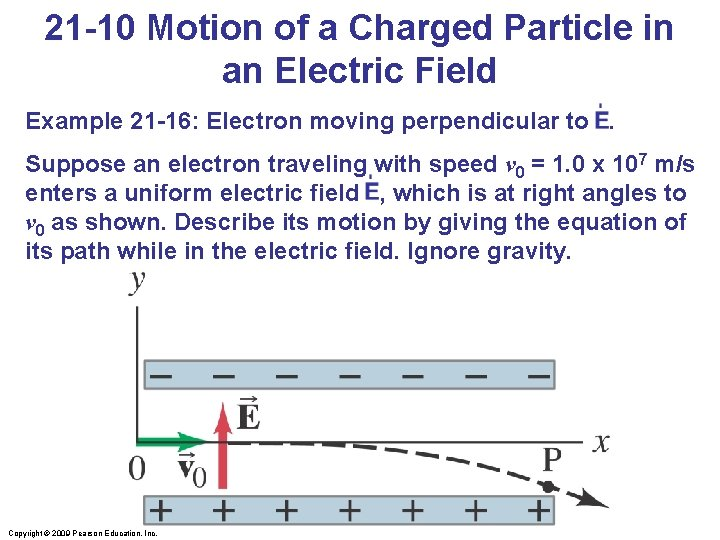21 -10 Motion of a Charged Particle in an Electric Field Example 21 -16: Electron moving perpendicular to. Suppose an electron traveling with speed v 0 = 1. 0 x 107 m/s enters a uniform electric field , which is at right angles to v 0 as shown. Describe its motion by giving the equation of its path while in the electric field. Ignore gravity. Copyright © 2009 Pearson Education, Inc.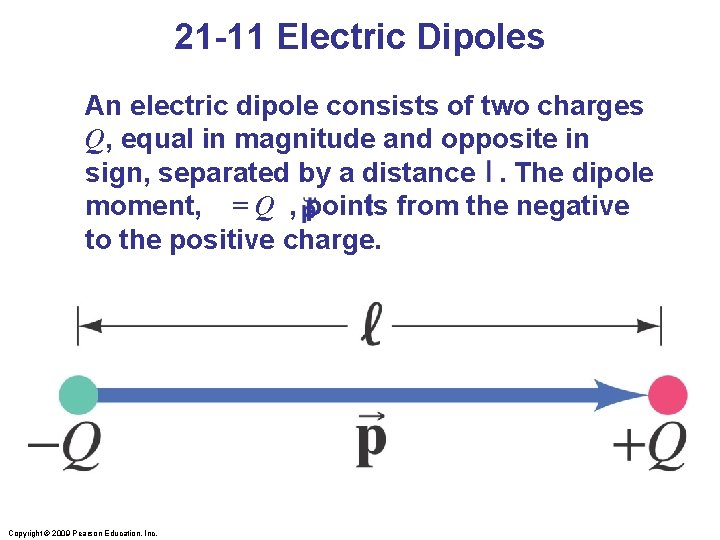21 -11 Electric Dipoles An electric dipole consists of two charges Q, equal in magnitude and opposite in sign, separated by a distance. The dipole moment, p = Q , points from the negative to the positive charge. Copyright © 2009 Pearson Education, Inc.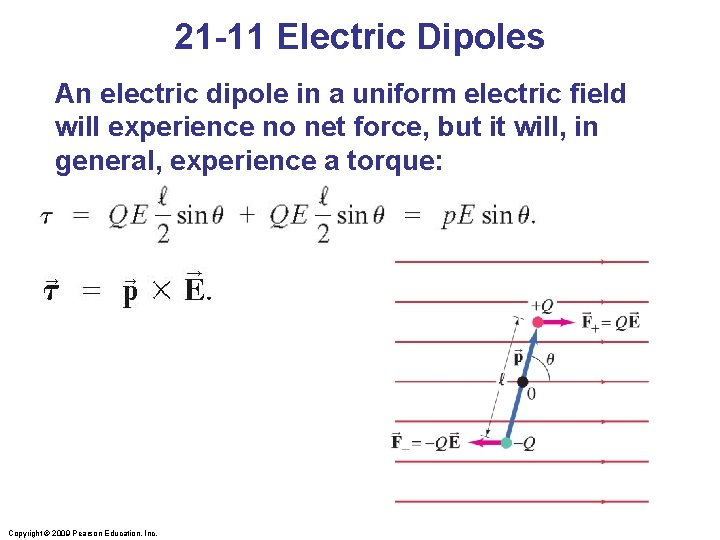21 -11 Electric Dipoles An electric dipole in a uniform electric field will experience no net force, but it will, in general, experience a torque: Copyright © 2009 Pearson Education, Inc.21 -11 Electric Dipoles The electric field created by a dipole is the sum of the fields created by the two charges; far from the dipole, the field shows a 1/r 3 dependence: Copyright © 2009 Pearson Education, Inc.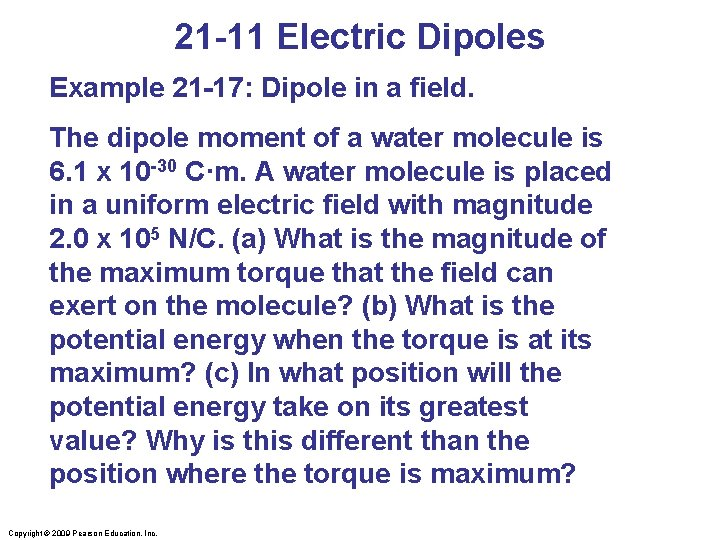21 -11 Electric Dipoles Example 21 -17: Dipole in a field. The dipole moment of a water molecule is 6. 1 x 10 -30 C·m. A water molecule is placed in a uniform electric field with magnitude 2. 0 x 105 N/C. (a) What is the magnitude of the maximum torque that the field can exert on the molecule? (b) What is the potential energy when the torque is at its maximum? (c) In what position will the potential energy take on its greatest value? Why is this different than the position where the torque is maximum? Copyright © 2009 Pearson Education, Inc.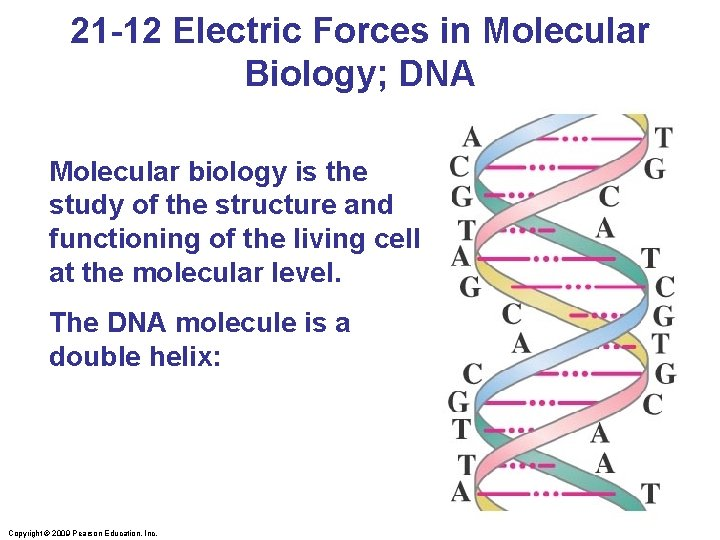21 -12 Electric Forces in Molecular Biology; DNA Molecular biology is the study of the structure and functioning of the living cell at the molecular level. The DNA molecule is a double helix: Copyright © 2009 Pearson Education, Inc.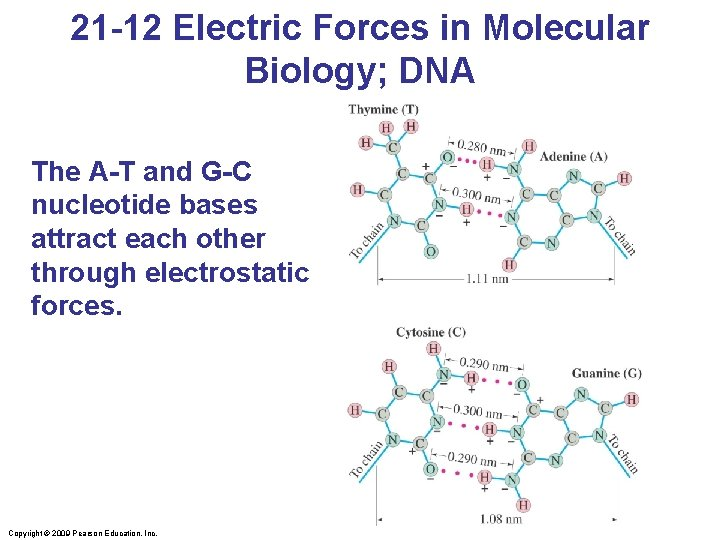21 -12 Electric Forces in Molecular Biology; DNA The A-T and G-C nucleotide bases attract each other through electrostatic forces. Copyright © 2009 Pearson Education, Inc.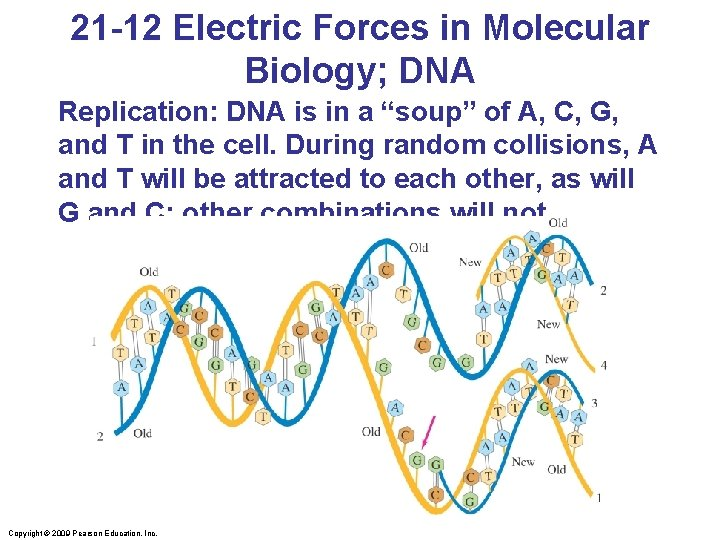21 -12 Electric Forces in Molecular Biology; DNA Replication: DNA is in a “soup” of A, C, G, and T in the cell. During random collisions, A and T will be attracted to each other, as will G and C; other combinations will not. Copyright © 2009 Pearson Education, Inc.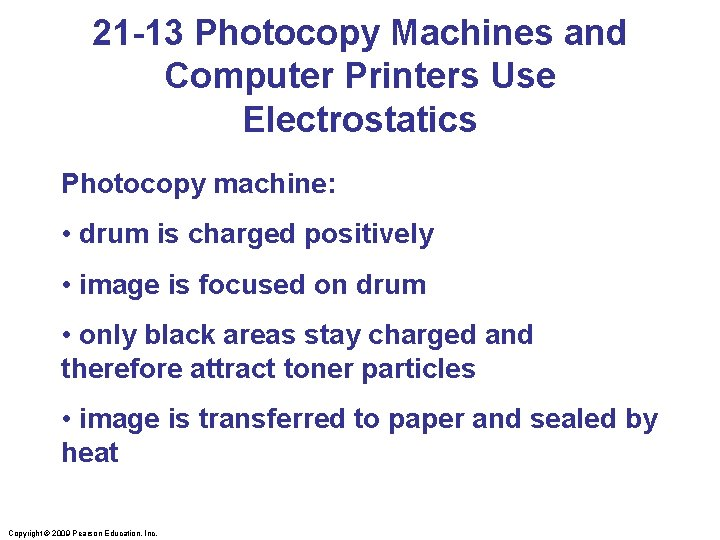21 -13 Photocopy Machines and Computer Printers Use Electrostatics Photocopy machine: • drum is charged positively • image is focused on drum • only black areas stay charged and therefore attract toner particles • image is transferred to paper and sealed by heat Copyright © 2009 Pearson Education, Inc.21 -13 Photocopy Machines and Computer Printers Use Electrostatics Copyright © 2009 Pearson Education, Inc.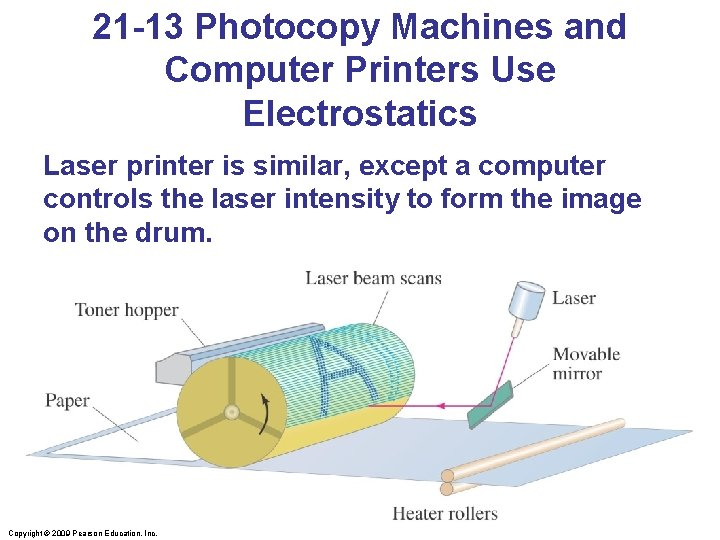21 -13 Photocopy Machines and Computer Printers Use Electrostatics Laser printer is similar, except a computer controls the laser intensity to form the image on the drum. Copyright © 2009 Pearson Education, Inc.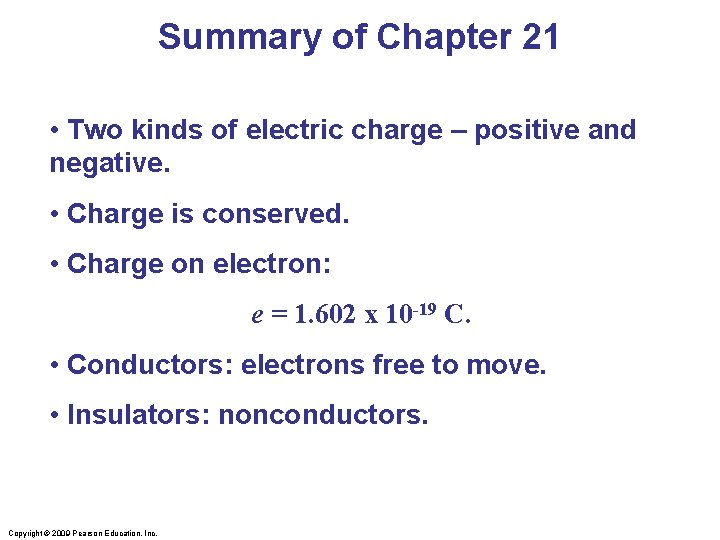Summary of Chapter 21 • Two kinds of electric charge – positive and negative. • Charge is conserved. • Charge on electron: e = 1. 602 x 10 -19 C. • Conductors: electrons free to move. • Insulators: nonconductors. Copyright © 2009 Pearson Education, Inc.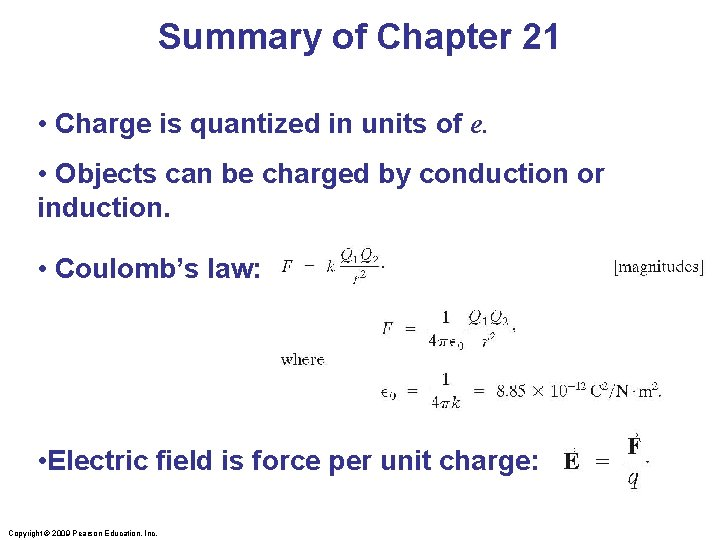Summary of Chapter 21 • Charge is quantized in units of e. • Objects can be charged by conduction or induction. • Coulomb’s law: • Electric field is force per unit charge: Copyright © 2009 Pearson Education, Inc.Summary of Chapter 21 • Electric field of a point charge: • Electric field can be represented by electric field lines. • Static electric field inside conductor is zero; surface field is perpendicular to surface. Copyright © 2009 Pearson Education, Inc.